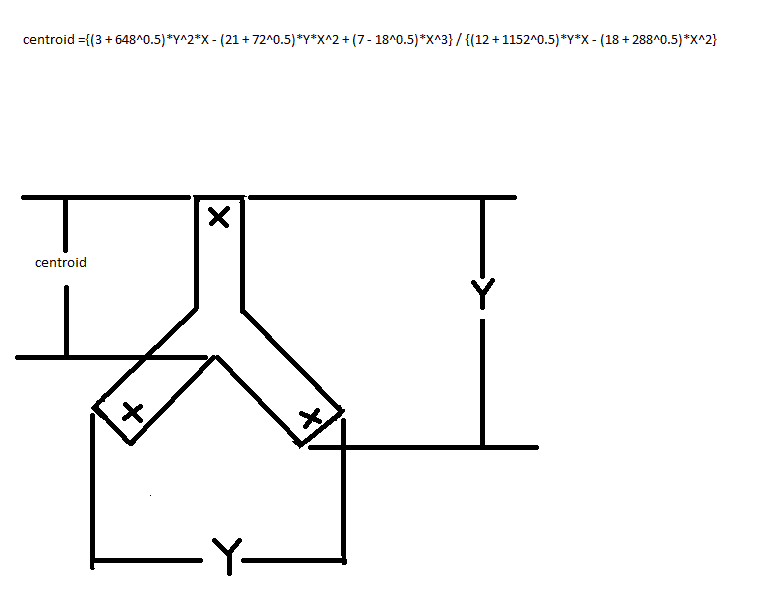# The Y/ X Ratio?

Geometry Level 3If the formula for this figure's perimeter $= ( 1 + 8^{0.5}) Y + ( 2 - 8^{0.5} ) X = 27.64$

and for this figure's area $= \dfrac {( 1 + 8^{0.5} ) Y X} 2 + \dfrac {X^2} { 128^{0.5} - 12} = 12.78$.

What does $\dfrac Y X$ equal?

Give your answer to 3 decimal places.

×

Problem Loading...

Note Loading...

Set Loading...